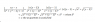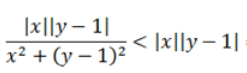# Help with Epsilon Delta Proof of Multivariable Limit

• joe5185
In order to show this, you need to use the substitution x = a and y = -1 in the original inequality, and then use the Chain Rule.The Attempt at a SolutionLooking at the answer I see that the limit does not exist; however when I do the epsilon delta proof I can't see where I went wrong because I keep getting the result that it does :( ? So I attached a picture detailing my argument and I would love for someone to tell me where I went wrong. The following inequality from your picture does not hold if x2 + (y - 1)2 < 1 . In order to show this, you need to use the substitution x = a and y = -1 in thef

## Homework Statement

Hey guys. I am having a little trouble answering this question. I am teaching myself calc 3 and am a little confused here (and thus can't ask a teacher). I need to find the limit as (x,y) approaches (0,1) of f(x,y) when f(x,y)=(xy-x)/(x^2+y^2-2y+1).

## Homework Equations

|f(x,y)-L|<epsilon
0<sqrt((x-a)^2+(y-b)^2))<delta

## The Attempt at a Solution

Looking at the answer I see that the limit does not exist; however when I do the epsilon delta proof I can't see where I went wrong because I keep getting the result that it does :( ? So I attached a picture detailing my argument and I would love for someone to tell me where I went wrong. I chose L in the epsilon delta definition to be 0 because this is what I get when I approach (0,1) along x=0, y=1, and y=x^3+1 . I am aware that the limit does not exist because if you travel along x=y^2-1 you get a value other than zero. However my only concern is why my logic is not correct in the attached image. Thanks a lot! Also if you have tips for doing these epsilon delta proofs I would love to hear them.

#### Attachments

•argument.png
9 KB · Views: 2,214
Last edited:
You can factor the numerator and get x(y-1) . Meanwhile your denominator factors and you get x^2+(y-1)^2. If you let ## x=\epsilon ## (it approaches zero) and let ## y-1=\Delta ## you then get a simple expression for the limit in terms of ## \epsilon ## and ## \Delta ##. If you let ## \Delta=\alpha \epsilon ## the result depends on ## \alpha ##. Thereby you don't have a single limit that it converges to. And I see your error=your denominator is greater than zero but it is not greater than 1.(top line=your inequality is incorrect.)

•joe5185
thank you so much. I completely follow you here:). Do you mind elaborating on when I can use the technique where you choose what in your expression is delta and what is epsilon? It seems pretty powerful but I just want to make sure when and how to use it. Thanks for the help

thank you so much. I completely follow you here:). Do you mind elaborating on when I can use the technique where you choose what in your expression is delta and what is epsilon? It seems pretty powerful but I just want to make sure when and how to use it. Thanks for the help
You have two variables, x, y that are approaching a,b respectively. Let ## x-a=\epsilon ## and ## y-b=\Delta ##. The ## \epsilon ## and the ## \Delta ## both approach zero, but there's nothing that says ## \epsilon=\Delta ##. You can let ## \Delta=\alpha \epsilon ##.The constant ## \alpha ## is quite arbitrary. If you could show your expression to give an answer that is independent of ## \alpha ##, then the limit would be what you computed by evaluating the expression with the ## \epsilon ## and ## \Delta ##. Hopefully this is helpful.

•## Homework Statement

Hey guys. I am having a little trouble answering this question. I am teaching myself calc 3 and am a little confused here (and thus can't ask a teacher). I need to find the limit as (x,y) approaches (0,1) of f(x,y) when f(x,y)=(xy-x)/(x^2+y^2-2y+1).

## Homework Equations

|f(x,y)-L|<epsilon
0<sqrt((x-a)^2+(y-b)^2))<delta